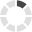## Change CountryLoading... Please wait...

#### Connect with us# Workplace Mathematics: Decimals

## Product Description

Workplace Mathematics: Decimals is part three of the Workplace Mathematics four-part training series.

Course objectives include:

• Learn about the use of decimals
• Learn the value of zeros in decimals
• Learn to round off decimals
• Learn to identify repeating decimals
• Learn to add, subtract, multiply, and divide decimals
• Learn to calculate percents.

Understanding Decimals

• Basics of Decimals

Working with Decimals

• Place Values
• Rounding Off Decimals

• Subtraction

Multiplying and Dividing Decimals

• Multiplication
• Division

Percentages

• Basics of Percentages
• Conversions

Workplace Mathematics: Introduction to Algebra is part three of the Workplace Mathematics three-part training series on DVO.

Course objectives include:

• Learn about signed numbers and how they are represented on a number line
• Learn to subtract, multiply, and divide signed numbers
• Learn to use variables in solving equations
• Learn to determine the value of square roots
• Learn to use numbers with exponents and powers of 10
• Learn to simplify algebraic expressions by removing grouping symbols
• Learn to perform operations in their proper sequence
• Learn to solve equations that have one unknown.

Our DVDs are portable and easy-to-use. Training content is "chapterized," or organized by learning objectives to:

• Enable training administrators to cover specific training points and conduct refresher training
• Facilitate classroom discussion.
Quantity:
Length:
SKU: MAT003

## Other Details

RUNTIME:
21
STRINTENG:
MAT003-INT-ENG/IS/